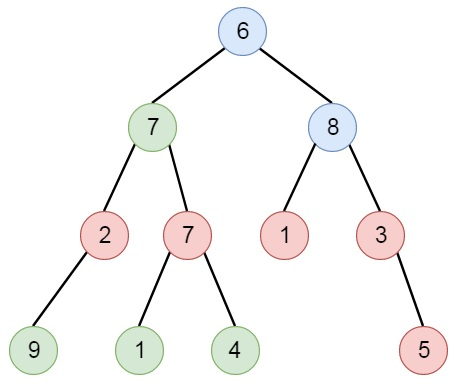# Sum of Nodes with Even-Valued Grandparent in C++

C++Server Side ProgrammingProgramming

Suppose we have a binary tree, we have to find the sum of values of nodes with even-valued grandparent. (A grandparent of a node is the parent of its parent, if it exists.). If there are no such nodes with an even-valued grandparent, then return 0. So if the tree is like −The output will be 18. The red nodes are nodes with even-value grandparent, while the blue nodes are the even valued grandparents.

To solve this, we will follow these steps −

• Define a map called parent
• Define a method called solve(), this will take node and par
• if node is null, then return
• if par is not null and par is present in parent and parent[par] is not 0 and value of parent[par] is even, then
• res := res + value of node
• parent[node] := par
• solve(left of node, node)
• solve(right of node, node)
• From the main method, set res := 0, call solve(root, Null), then return res

## Example

Let us see the following implementation to get better understanding −

class Solution {
public:
int res;
map <TreeNode*, TreeNode*> parent;
void solve(TreeNode* node, TreeNode* par = NULL){
if(!node)return;
if(par && parent.count(par) && parent[par] && parent[par]->val % 2 == 0){
res += node->val;
}
parent[node] = par;
solve(node->left, node);
solve(node->right, node);
}
int sumEvenGrandparent(TreeNode* root) {
res = 0;
parent.clear();
solve(root);
return res;
}
};

## Input

[6,7,8,2,7,1,3,9,null,1,4,null,null,null,5]

## Output

18